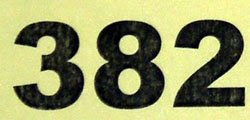## Monday, June 29, 2009

### 382

382 = 2 x 191.

382 is the maximum number of regions into which 20 circles divide the plane.

382 is the sum of ten consecutive primes: 19 + 23 + 29 + 31 + 37 + 41 + 43 + 47 + 53 + 59 = 382.

382 is a Smith number.

The sum of the divisors of 382 is a square: 1 + 2 + 191 + 382 = 576 = 242.382 Dodona is a large main-belt asteroid discovered in 1894.

Source:
Wikipedia